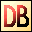DelphiBasics
 CompareValueFunction Compare numeric values with a tolerance Math unit
 1 function CompareValue(const A, B Integer|Int64):TValueRelationship; 2 function CompareValue (const A, B Single|Double|Extended; delta Single|Double|Extended:TValueRelationship;
Description
CompareValue allows floating point numbers to be compared in a tolerant way. If the two numbers are close enough together, they are deemed to be equal. The delta value is the permitted tolerance.

There seems no point to the author in providing the Integer version of this function with no delta.

TValueRelationship supports the following values :
 LessThanValue -1 EqualsValue 0 GreaterThanValue 1
Related commands
 Max Gives the maximum of two integer values Min Gives the minimum of two integer values Mean Gives the average for a set of numbersDownload this web site as a Windows program.

 Example code : Comparing floating point numbers var   A : Single;   B : Single;   C : Single; begin   A := 23.0;   B := 23.0;   C := 23.1;   // Compare 2 equal floats   case CompareValue(A, B) of     LessThanValue    : ShowMessage('A < B');     EqualsValue      : ShowMessage('A = B');     GreaterThanValue : ShowMessage('A > B');   end;   // Compare 2 unequal floats   case CompareValue(A, C) of     LessThanValue    : ShowMessage('A < C');     EqualsValue      : ShowMessage('A = C');     GreaterThanValue : ShowMessage('A > C');   end;   // Compare 2 unequal floats - but allow for a difference of up to +/- 0.2   case CompareValue(A, C, 0.2) of     LessThanValue    : ShowMessage('A < C');     EqualsValue      : ShowMessage('A = C');     GreaterThanValue : ShowMessage('A > C');   end; end; Show full unit code A = B   A < C   A = C